SSC  >  Logical Reasoning - Test 6

# Logical Reasoning - Test 6

Test Description

## 25 Questions MCQ Test SSC CGL Tier 1 Mock Test Series | Logical Reasoning - Test 6

Logical Reasoning - Test 6 for SSC 2022 is part of SSC CGL Tier 1 Mock Test Series preparation. The Logical Reasoning - Test 6 questions and answers have been prepared according to the SSC exam syllabus.The Logical Reasoning - Test 6 MCQs are made for SSC 2022 Exam. Find important definitions, questions, notes, meanings, examples, exercises, MCQs and online tests for Logical Reasoning - Test 6 below.
Solutions of Logical Reasoning - Test 6 questions in English are available as part of our SSC CGL Tier 1 Mock Test Series for SSC & Logical Reasoning - Test 6 solutions in Hindi for SSC CGL Tier 1 Mock Test Series course. Download more important topics, notes, lectures and mock test series for SSC Exam by signing up for free. Attempt Logical Reasoning - Test 6 | 25 questions in 15 minutes | Mock test for SSC preparation | Free important questions MCQ to study SSC CGL Tier 1 Mock Test Series for SSC Exam | Download free PDF with solutions
 1 Crore+ students have signed up on EduRev. Have you?
Logical Reasoning - Test 6 - Question 1

### Identify the diagram that best represents the relationship among the classes given. Woman, politician, smart

Detailed Solution for Logical Reasoning - Test 6 - Question 1

Clearly, all the three are interrelated. Some women may be politicians and some may be smart. Similarly, some politicians may be smart and may be women.
So, all the three circles intersect with each other.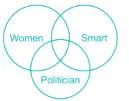Therefore, above diagram best express relationship between Woman, politician, smart

Logical Reasoning - Test 6 - Question 2

### Arrange the given words in alphabetical order and tick the one that comes in the middle Remove, rumour, rigour, revive, retrospect

Detailed Solution for Logical Reasoning - Test 6 - Question 2

These words can be properly arranged as:
remove, retrospect, revive, rigour, rumour
clearly, the middle word is revive.

Logical Reasoning - Test 6 - Question 3

### Pointing to a boy a woman says, “His father is father – in – law of that person whose father is father – in – law of mine”. Then how boy is related to the woman?

Detailed Solution for Logical Reasoning - Test 6 - Question 3

Table is drawn describing symbols and their meanings: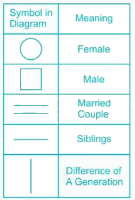“His father is father – in – law of that person whose father is father – in – law of mine”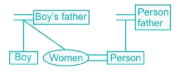Hence, Boy is a Brother of Women.

Logical Reasoning - Test 6 - Question 4

Which of the following words would correctly decode the word ZHOFRPH if the simple alphabet shifting code is used?

Detailed Solution for Logical Reasoning - Test 6 - Question 4

Here only simple alphabet shifting code is used
Here if we look on code word “ZHOFRPH” then we can see that for given word second and last letter are same therefore on decoding we will get a word which will be having 2nd letter and last letter same.
Now if we look on given option we will be find that that word “WELCOME” will be having 2nd letter and last letter same
Now we can see that letter shifting as given in below figure.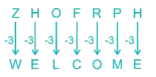Hence, on decoding “ZHOFRPH” we will get the word “WELCOME”

Logical Reasoning - Test 6 - Question 5

In this question, the sets of letter given in the alternatives are represented. The columns and rows of Matrix I are numbered from J to N. A number from these matrices can be represented first by its row and next by its column, e.g., ‘1’ can be represented by KM, NN etc., and '2' can be represented by LM, NM, etc. Similarly you have to identify the set for the letter for number ‘78269’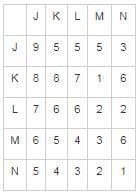Detailed Solution for Logical Reasoning - Test 6 - Question 5

1) KL, KK, NL, LL, JJ ⇒ 7, 8, 3, 6, 9
2) KL, ML, LM, LL, JJ ⇒ 7, 4, 2, 6, 9
3) KL, KK, LM, LL, JK ⇒ 7, 8, 2, 6, 5
4) KL, KK, LM, LL, JJ ⇒ 7, 8, 2, 6, 9
Therefore, “KL, KK, LM, LL, JJ” are set of letter which represent number“78269”

Logical Reasoning - Test 6 - Question 6

Select the related word from the given alternatives.

Insane : sensible ∷ ?

Detailed Solution for Logical Reasoning - Test 6 - Question 6

→ Insane means mad, opposite of sensible, likewise climb is opposite of Descend.

Logical Reasoning - Test 6 - Question 7

Select the related letters from the given alternatives.

BCD : DEG : : FIJ : ?

Detailed Solution for Logical Reasoning - Test 6 - Question 7

We know that position of letter in english alphabet
⇒ B = 2, C = 3, D = 4
BCD = 2 + 3 + 4 = 9 = 32
⇒ D = 4, E = 5, G = 7
DEG = 4 + 5 + 7 = 16 = 42
Similarly,
⇒ F = 6, I = 9, J = 10
FIJ = 6 + 9 + 10 = 25 = 52
⇒ J = 10, L = 12, N = 14
JLN = 10 + 12 + 14 = 36 = 62
Hence, “JLN” is correct answer.

Logical Reasoning - Test 6 - Question 8

Directions: In the following question, select the related number from the given alternatives.

392 : 28 :: 722 :  ?

Detailed Solution for Logical Reasoning - Test 6 - Question 8

392/28 = 14 = half of 28
722/38 = 19 = half of 38.
Hence, correct answer is 38.

Logical Reasoning - Test 6 - Question 9

Select the odd word from the given alternatives.

Detailed Solution for Logical Reasoning - Test 6 - Question 9

→ Except Bride and Groom, all others options pointing to husband and wife with legal commitment. Even Jack and Jill are considered to represent a married royal couple(Louis XVI of France and  his Queen Marie Antoinette)

Logical Reasoning - Test 6 - Question 10

Select the one which is different from the other three responses.

Detailed Solution for Logical Reasoning - Test 6 - Question 10

The pattern followed is that in each term the difference between 1st& 2nd and 3rd& 4th term is same i.e.
In YDWB→ Y + 5 = D; W + 5 = B
TKRI→ T – 9 = K; R – 9 = I
QNON→ Q – 3 = N; O – 1 = N
HLFJ→ H + 4 = L; F + 4 = J
Clearly, QNON is odd one.

Logical Reasoning - Test 6 - Question 11

In the given series one term is missing. Select the correct alternative from the given ones that will complete the series.

XOXXX, XXOXX, XXXOX, XXXXO, _________

Detailed Solution for Logical Reasoning - Test 6 - Question 11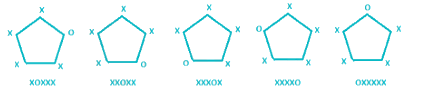→ ‘O’ is rotating in clockwise cyclic order.

Logical Reasoning - Test 6 - Question 12

A series is given with one term missing. Select the correct alternative from the given ones that will complete the series.
Red, Orange, Yellow, ?

Detailed Solution for Logical Reasoning - Test 6 - Question 12

→ In Colour spectrum the sequence of wavelength is as follow :
Red (780 to 622 nm)
Orange (622 to 597 nm)
Yellow (597 to 577 nm)
Green (577 to 492 nm)
→ In above sequence next colour will be Green.

Logical Reasoning - Test 6 - Question 13

In the given series one number is missing. Select the correct alternative from the given ones that will complete the series.

7, 3, _, -2, -3​

Detailed Solution for Logical Reasoning - Test 6 - Question 13

→ Sequence is like this and follows following rule: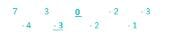Therefore, next term in series will be “0”.

Logical Reasoning - Test 6 - Question 14

In the following question, which one set of letters when sequentially placed at the gaps in the given letter series shall complete it?

r_pr_p_q_r_p

Detailed Solution for Logical Reasoning - Test 6 - Question 14

→ In above sequence there is repetition of ‘rqp’ and sequence is like this rqp rqp rqp rqp.
Therefore, “qqrpq” are group of letter which will complete the given series.

Logical Reasoning - Test 6 - Question 15

Two football players A and B start from the same point. A runs 10m North, then 15 m East, turns right and runs 25 m. Meanwhile, B runs 7 m West, then 15 m South, turns left and runs 2 m. What is the position of B with respect to A?

Detailed Solution for Logical Reasoning - Test 6 - Question 15

Direction diagram can be traces as shown below,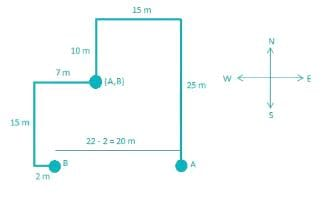→ From above diagram, B is 20 m to the West of A.

Logical Reasoning - Test 6 - Question 16

In the question one statement is given, followed by two arguments, I and II. You have to consider the statement to be true even if it seems to be at variance from commonly known facts. You have to decide which of the given arguments, if any, is a strong argument.

Statement: Should the village authorities build a water tank in their village?
Argument I: Yes, a tank is essential as it will provide water for dry summer months.
Argument II: No, the sharing of tank water will lead to disharmony amongst the villagers.

Detailed Solution for Logical Reasoning - Test 6 - Question 16

→ Argument I is strong, If village authorities are building a water tank in village, means it’s necessary and essential for village. Sharing water lead to disharmony amongst the villagers cannot be strong argument because first we have to think about essential thing that is build water tank for villagers, so argument I is strong.

Logical Reasoning - Test 6 - Question 17

Which of the cubes in the given options can't be made from the below unfolded cube?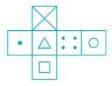Detailed Solution for Logical Reasoning - Test 6 - Question 17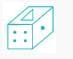→ Option 4th cube is not possible from unfolded cube.

Logical Reasoning - Test 6 - Question 18

Select the correct pattern from the options that can be combined to make the given figure.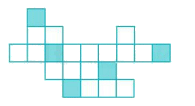Detailed Solution for Logical Reasoning - Test 6 - Question 18

→ Combining figures of 1st option: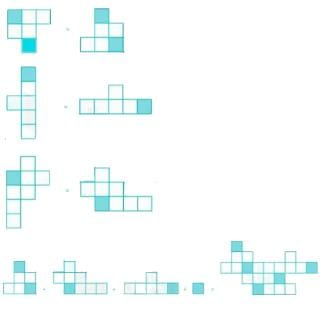→ Option 1st is correct.

Logical Reasoning - Test 6 - Question 19

A piece of paper is folded and punched as shown below in the question figures. From the given answer figures, indicates how it will appear when opened.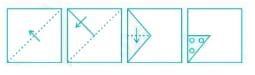Detailed Solution for Logical Reasoning - Test 6 - Question 19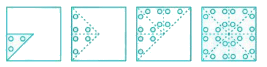→ After opening paper, paper look like 2nd figure, so 2nd option is correct.

Logical Reasoning - Test 6 - Question 20

If mirror is placed on the line MN, then which of the answer figures is the right of the given figure?Detailed Solution for Logical Reasoning - Test 6 - Question 20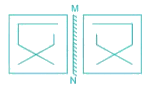Above figure represent correct mirror image of given question figure.

Logical Reasoning - Test 6 - Question 21

If o < l, x < o, a < l and p < o, which of the following must be true?

I. a > p
II. l > p
III. x < l

Detailed Solution for Logical Reasoning - Test 6 - Question 21

I. a > p
For this inequality, first we have to find relationship between ‘a’ and ‘o’.
Given that, a < l and o < l but we cannot determine relationship between ‘a’ and ‘o’ from above inequalities.
So, a > p is not decidable.
II. l > p
Given that, o < l and p < o
From above two inequalities we can say p <
III. x < l
Given that, o < l and x < o
From above two inequalities we can say x <
→ Only II and III are true, option 4th is correct.

Logical Reasoning - Test 6 - Question 22

If '+' is 'x', '-‘ is '+', 'x' is '÷' and '÷' is '-‘, then answer the following question based on this information.

13.5 x 1.5 - 0.008 + 125 = ?

Detailed Solution for Logical Reasoning - Test 6 - Question 22

→ After applying above conditions:
= 13.5 ÷ 1.5 + 0.008 x 125
= (13.5 ÷ 1.5) + (0.008 x 125)
= 9 + 1
= 10

Logical Reasoning - Test 6 - Question 23

In the following question, select the missing number from the given alternatives.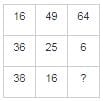Detailed Solution for Logical Reasoning - Test 6 - Question 23

Here, if we observe it carefully, then we can see that sum number of each column is 90.
16 + 36 + 38 = 90
49 + 25 + 16 = 90
64 + 6 + ? = 90
? = 90 – 70 = 20
Therefore, “20” will be the missing term.

Logical Reasoning - Test 6 - Question 24

Directions: In question, two statements are given followed by conclusion. You have to consider the statement to be true even if it seems to be at variance from commonly known facts. You are to decide which of the given conclusion can definitely be drawn from the given statement. Indicate your answer.

Statements:
(I) Some students are intelligent.
(II) Ankita is a student.

Conclusions:
1. Some students are dull.
2. Ankita is intelligent.

Detailed Solution for Logical Reasoning - Test 6 - Question 24

Least possible Venn diagram for the given statement will be,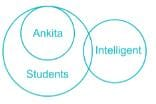Conclusions:
1. Some students are dull → In statement nothing is mentioned about dull. So we cannot say ‘Some statement are dull’. Hence false.
2. Ankita is an intelligent → This may be possible but not definite. Hence false.
Hence none of the conclusions follows.

Logical Reasoning - Test 6 - Question 25

Amit, Bhuwan, Chetan, Lalit, Dilip and Fahim are sitting in a row facing west. Chetan is between Amit and Lalit. Bhuwan is just to the left of Lalit but right of Dilip. Chetan sitting to the immediate right of Lalit. Fahim is at the extreme left end. Which pair is sitting by the sides of Dilip?

Detailed Solution for Logical Reasoning - Test 6 - Question 25

Chetan is between Amit and Lalit. Bhuwan is just to the left of Lalit but right of Dilip.
Chetan sitting to the immediate right of Lalit. Fahim is at the extreme left end.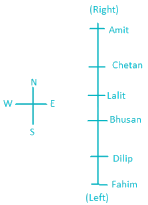Hence, Bhusan and Fahim is sitting by the sides of Dilip.

## SSC CGL Tier 1 Mock Test Series

42 docs|103 tests
 Use Code STAYHOME200 and get INR 200 additional OFF Use Coupon Code
Information about Logical Reasoning - Test 6 Page
In this test you can find the Exam questions for Logical Reasoning - Test 6 solved & explained in the simplest way possible. Besides giving Questions and answers for Logical Reasoning - Test 6, EduRev gives you an ample number of Online tests for practice

## SSC CGL Tier 1 Mock Test Series

42 docs|103 tests